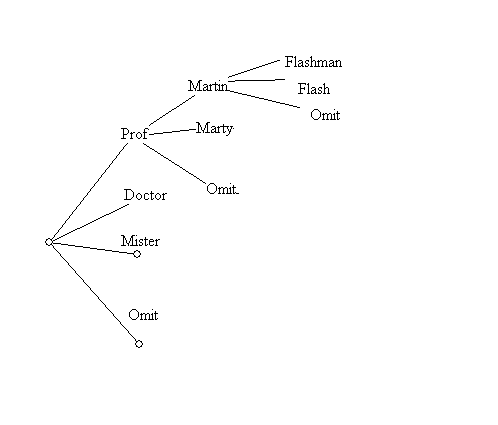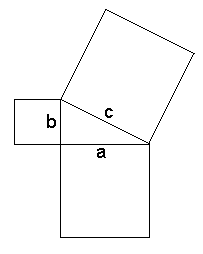Thursday, January 26

• More Introduction to course organization.
• Examples for Portfolios and Projects.
• Web Materials and Notes.
• Why focus on Visual Mathematics?
• Mathematics that studies topics related to visual experience. [Geometry, Topology, Motion]
• Visualization of mathematics that is not inherently visual. [Visualizing Counting]
• Classification:  Triangles, circles: congruence and  similarity.  What common features are shared by circles and triangles?... They divide the plane into two sectors: "inside and outside". Not in the same class as the symbol.  8.

• Example of "visual Math" and counting:
• My Name: Martin Flashman
• How to start a letter to me:  Hi ___________
•  Professor Martin Flashman Doctor Marty Flash Mister Omit Omit Omit
• How many different openings are possible?
• We can visualize this problem with a "tree"• This visualization allows us to count the possibilities easily...

• seeing there are 9 possibilities for each of 4 title branches
so that the total is 9*4 = 36 possibilities.
• There were several alternative countings suggested for this problem. This may be connected to some ambiguity in the statement of what would be an acceptable opening for the letter. So- if the assumption is made that some entry must be made then the choice of an omission for each selection would be eliminated from the tally, giving 25 possibilities.  A distinction:
• "vague" meaning "Not clear in meaning or application."  and
• "ambiguious" meaning "Open to more than one interpretation. Ambiguous indicates the presence of two or more possible meanings."
• This is an example of a visualization used to understand and solve a problem that initially is not connected to anything visual .
• What is Visual Mathematics?
• Mathematics that studies topics related to visual experience. [Geometry, Topology, Motion]
• Visualization of mathematics that is not inherently visual. [Visualizing Counting]
• Example: Numbers...
• numeral: a symbol for representing a number
• Number: a form of universal language to describe anything/ physical things/ concepts related to measurement
• such as V, 5, five, cinq, chamesh, cinco
• Frege distinguished numerals from numbers in the late 19th century.
• We can compare numbers... for instance we say" 3 is less than 5"
• Is 3 smaller than 5?
• Numerals are symbols (visual or linguistic) that we use to represent numbers.
• We use numbers to measure (lengths) and put things in order (which was first).
• Another common visual representaion of numbers uses the number line.
• ___.___.___.___.___.___.___.______
•       1     2     3     4     5     6     7

• Here the numerals are associated with points, so the points are considered to visualize the corresponding numbers.

• We visualize equations that give relations between numbers with graphs in the coordinate plane.

• 3x + 2y = 6 is visualized by the graph of a line.

• Miscellaneous: Some topics we will study.
• The film lists as a guide to the course topics.
• Symmetry.(Chapter 5)
• Tiling. (Chapter 5)
• Similarity and Projection. (Chapter 4)
• The color problem. (Chapter 6)
• The motion problem.(Chapter 3)
• The Sphere and the Torus. Surfaces (Chapter 6)

Theme Question:Who first showed the earth was a sphere?

• Discussion on the The Sphere  and the Torus. [to be continued next class]

• Question 1. How can one distinguish the sphere from a plane (Flatland) based solely on experiences on the surface?

Question 2. How can one distinguish the sphere from a torus based solely on experiences on the surface?

• Measurement and the Pythagorean Theorem (PT)
•a2 + b2 = c2

Show video on PT.
Outline:
Area of triangles = 1/2 bh
Area of parallelogram= bh
Scaling: a linear scale change of r gives area change of factor r2.
3 questions: running, moat, wind power...
Proof of the PT: Similar right triangles: c= a2 /c + b2 /c .

To be continued next class!